# ISEE Middle Level Math : How to find the decimal equivalent of a fraction

## Example Questions

### Example Question #171 : How To Find The Decimal Equivalent Of A Fraction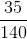is equivalent to which of the following?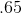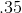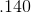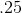Explanation:

To convert a fraction to a decimal, divide the numerator (top half) by the denominator (bottom half):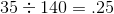Answer:### Example Question #172 : How To Find The Decimal Equivalent Of A Fraction

How do you write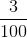as a decimal?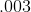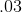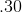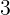Explanation:

Divide the numerator (top half of the fraction) by the denominator (bottom half of the fraction) to convert the fraction to a decimal: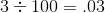Answer:### Example Question #173 : How To Find The Decimal Equivalent Of A Fraction

Write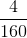as a decimal.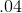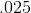Explanation:

Divide the numerator (top half of the fraction) by the denominator (bottom half of the fraction) to convert the fraction to a decimal: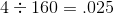Answer:### Example Question #174 : How To Find The Decimal Equivalent Of A Fraction

What is the decimal equivalent to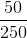?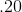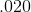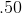Explanation:

Divide the numerator (top half of the fraction) by the denominator (bottom half of the fraction) to convert the fraction to a decimal: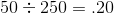Answer:### Example Question #175 : How To Find The Decimal Equivalent Of A Fraction

Convert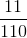to a decimal.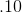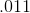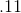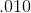Explanation:

Divide the numerator (top half of the fraction) by the denominator (bottom half of the fraction) to convert the fraction to a decimal: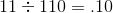Answer:### Example Question #171 : How To Find The Decimal Equivalent Of A Fraction

Arrange these numbers from least to greatest: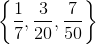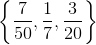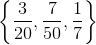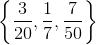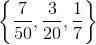Explanation:

Rewrite all three numbers as their decimal equivalents by dividing numerator by  denominator.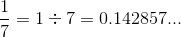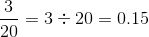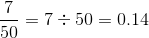From the decimal equivalents, the fractions can be arranged as follows:### Example Question #177 : How To Find The Decimal Equivalent Of A Fraction

Write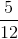as a decimal.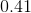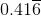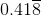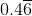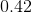Explanation:

Divide 5 by 12.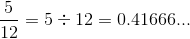Note that the "6" repeats forever. This can be written as.

### Example Question #178 : How To Find The Decimal Equivalent Of A Fraction

How many of the following four numbers are elements of the set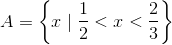(A)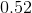(B)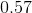(C)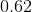(D)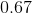Three

One

Four

None

Two

Three

Explanation: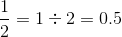and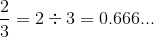, so we choose the numbers that fall between these two. Of the four choices, only 0.67 is not between these two numbers. The correct response is "three".

### Example Question #179 : How To Find The Decimal Equivalent Of A Fraction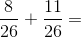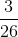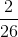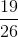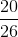Explanation:

Add the numerators and keep the denominator the same: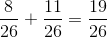Answer:### Example Question #171 : How To Find The Decimal Equivalent Of A Fraction

Arrange these numbers from least to greatest: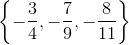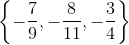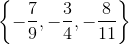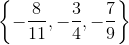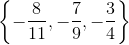Explanation:

Rewrite all three numbers as their decimal equivalents by dividing numerator by denominator.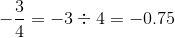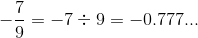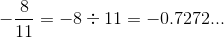To arrange negative numbers from least to greatest, we must arrange them so that their absolute values (values without the negative symbol) go from greatest to least. In descending order, the absolute values are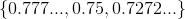,

so, in ascending order, the numbers themselves are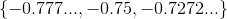or, in their fractional equivalents,.

### All ISEE Middle Level Math Resources